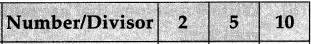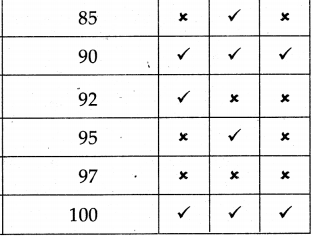# Maharashtra Board Class 5 Maths Solutions Chapter 8 Multiples and Factors Problem Set 33

Balbharti Maharashtra Board Class 5 Maths Solutions Chapter 8 Multiples and Factors Problem Set 33 Textbook Exercise Important Questions and Answers.

## Maharashtra State Board Class 5 Maths Solutions Chapter 8 Multiples and Factors Problem Set 33

Question 1.
(1) Write five three-digit numbers that are multiples of 2.
100, 102, 104, 106, 108.(2) Write five three-digit numbers that are multiples of 5.
100, 105, 110, 115, 120.

(3) Write five three-digit numbers that are multiples of 10.
100, 110, 120, 130, 140.

Question 2.
Write 5 numbers that are multiples of 2 as well as of 3.
2 as well as of 3 means 2 and 3 that is multiples of 6.
They are 6, 12, 18, 24, 30.

Question 3.
A ribbon is 3 metres long. Can we cut it into 50 cm pieces and have nothing left over? Write the reason why or why not.
3 metres = 300 cm.
We can cut it into 50 cm pieces.
Since 300 is exactly divisible 50.
That is 300 is multiples of 50.
300 ÷ 50 = 6
We will get 6 pieces, nothing is left over.Question 4.
A ribbon is 3 metres long. I need 8 pieces of ribbon each 40 cm long. How many centimetres shorter is the ribbon than the length I need?
1 piece of 40 cm, so for 8 pieces ribbon needed is 40 x 8 = 320 cm.
But ribbon is 3 metre = 300 cm long.
So ribbon is shorter by 320 – 300 = 20 cm.

Question 5.
If the number given in the table is divisible by the given divisor, put ✓ in the box. If it is not divisible by the divisor, put ✗ in the box.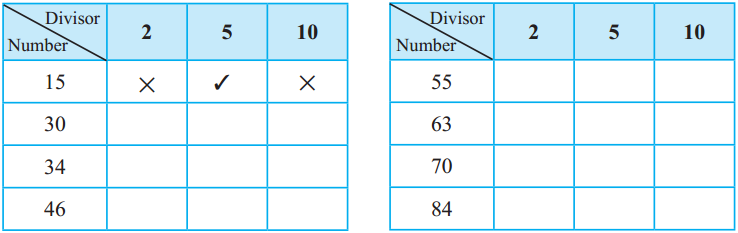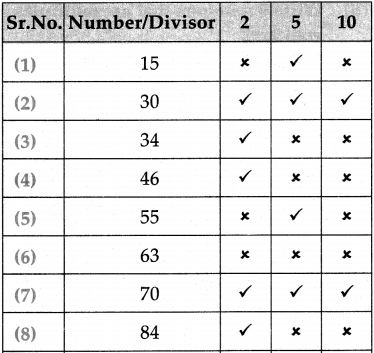Prime and composite numbers

Some numbers are given in the tables below. Write all of their factors.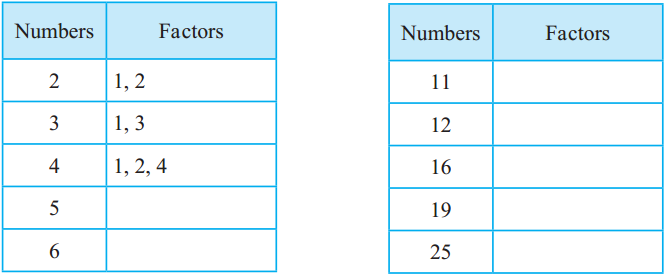Dada : What do you notice on studying the table?

Ajay : The number 1 is a factor of every number. Some numbers have only 1 and the number itself as factors. For example, the only factors of 3 are 1 and 3. Similarly, the factors of 2 are only 1 and 2 and the factors of 19 are only 1 and 19. Some numbers have more than two factors.

Dada : Numbers like 2, 3, 19 which have only two factors are called prime numbers.

A number which has only two factors, 1 and the number itself, is called a prime number.

Ajay : What do we call numbers like 4, 6 and 16 which have more than two factors?

Dada : Numbers like 4, 6 and 16 are called composite numbers.

A number which has more than two factors is called a composite number.

Dada : Think carefully and tell me whether 1 is a prime or composite number.

Ajay : The number 1 has only one factor, 1 itself, so I can’t answer your question.

Dada : You’re right. 1 is considered neither a prime number nor a composite number.

1 is a number which is neither prime nor composite.Question 1.
Write five three-digit numbers that are multiples of 3.
102, 105, 108, 111, 114.

Question 2.
Write five two-digit numbers that are multiples of 7.
14, 21, 28, 35, 42

Question 3.
Write five three-digit numbers that are multiples of 4.
112, 116, 120, 124, 128.

Question 4.
Write 5 numbers that are multiples of 3 as well as 5.
3 as well as 5 means 3 and 5. i.e. multiples of 15.
They are 15, 30, 45, 60, 75.Question 5.
A string is 4 metres long. Can we cut it into 50 cm pieces and have nothing left over?
4 metres = 400 cm.
We can cut it into 50 cm pieces.
Since 400 is exactly divisible by 50.
That is 400 is multiple of 50 400 + 50 = 8
We will get 8 pieces. Nothing is left over.

Question 6.
A paper Is 2 metres long. I need 8 pieces of paper each 30 cm long. How many centimetres shorter is the paper than the length I need?